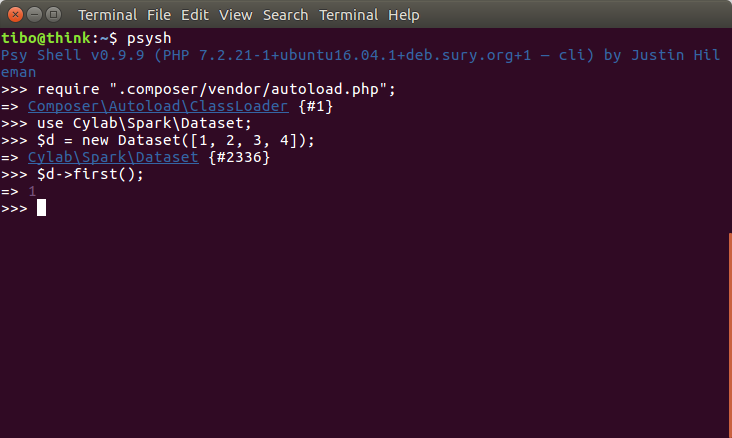# Apache Spark MapReduce with PHP

Aug 19, 2019 by Thibault Debatty | 3644 views

When it comes to Big Data processing, I'm a huge fan of the Apache Spark project. Spark is a very powerful tool to analyse very large datasets in parallel, and at the same time it provides a nice API that allows to write clean distributed code.

``````JavaRDD<String> lines = sc.textFile("data.txt");
JavaRDD<Integer> lineLengths = lines.map(s -> s.length());
int totalLength = lineLengths.reduce((a, b) -> a + b);``````

Sadly enough, there is currently no PHP driver to run PHP code on a Spark cluster.

However, to process smaller datasets, you can use php-spark. This library is a wrapper around (local) arrays that implements the same methods and syntax as Apache Spark. If you are used to Spark, this library will allow you to write PHP MapReduce code in a breeze!

``````\$data = new Dataset([1, 2, 3, 4]);
\$result = \$data
->map(function (\$v) {
return 2 * \$v;
})
->reduce(function (\$v, \$agg) {
return \$agg + \$v;
});

\$result == 20;``````

## Installation

Easiest way to install is using composer:

``composer require cylab/php-spark``

## Usage

The main component of the library is the Dataset. To create a dataset, simply pass an array of data to the constructor:

``````use CylabSparkDataset;

\$d = new Dataset([1, 2, 3, 4]);``````

A dataset is immutable, like a Resilient Distributed Dataset in Spark. Each operation creates a new Dataset:

``\$d2 = \$d->map(function (\$v) { return 2 * \$v; });``

Like in Spark, some methods expect a dataset containing <key, value> tuples, like reduceByKey(func)

``````use CylabSparkDataset;
use CylabSparkTuple;

\$strings = ["foe", "bar", "foe"];
\$d = new Dataset (\$strings);
\$d2 = \$d->map(function(\$s) { return new Tuple(\$s, 1); });

\$counts = \$d2->reduceByKey(function (\$count, \$sum) {
return \$sum + \$count;
});

# Tuple<"foe", 2>
var_dump(\$counts->first());``````

The complete list of methods and documentation is available on the website of the project: https://gitlab.cylab.be/tibo/php-spark

## Interactive shell

I really like to use php-spark with an interactive PHP shell, like psysh....

To install psysh:

``composer global require psy/psysh:@stable``

Install php-spark globally aswell:

``composer global require composer require cylab/php-spark``

Rebuild the global autoloader of composer:

``composer global dump-autoload``

You can now use php-spark interactively: